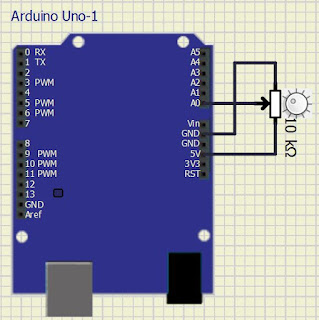# How to read the Arduino ADC value stable and reduce noise

How to read ADC Arduino becomes stable reducing noise - in certain applications, the ADC becomes very important. Reading a stable value will affect the final results of the reading.

Like the example in the following image using a 10k potentiometer connected to the A0 analog pin.Wiring ADC Arduino

Please use the circuit above, turn the potentiometer to the middle and leave position, the results of the ADC will be found that the final score sometimes changes like 515, 516, 517, 515, 517 and so on.

How do we make this change not happen too? The answer is with a smooting technique. So, the program will be made to read the ADC value for 10 times and from 10 times it will be taken on average.

The progam is as follows:

```int adc = 0;
const int jumlah_baca = 10;
int baca[jumlah_baca];
int jumlahArray = 0;
int nilai = 0;

void setup()
{
Serial.begin(9600);
}

void loop()
{
nilai = nilai - baca[jumlahArray];
nilai = nilai + baca[jumlahArray];
jumlahArray = jumlahArray + 1;

if (jumlahArray >= jumlah_baca) {
jumlahArray = 0;
}

adc = nilai / jumlah_baca; //get average value